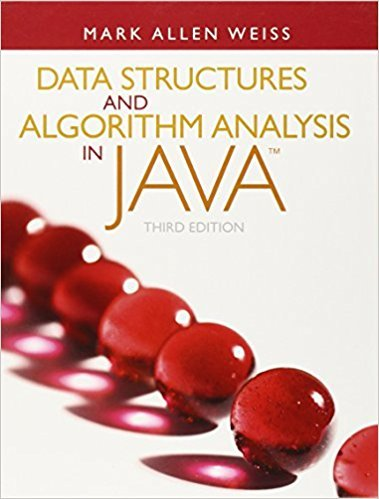×
Get Full Access to Data Structures And Algorithm Analysis In Java - 3 Edition - Chapter 9 - Problem 9.56
Get Full Access to Data Structures And Algorithm Analysis In Java - 3 Edition - Chapter 9 - Problem 9.56

×

# The baseball card collector problem is as follows: Given packets P1, P2, ... , PMISBN: 9780132576277 316

## Solution for problem 9.56 Chapter 9

Data Structures and Algorithm Analysis in Java | 3rd Edition

• Textbook Solutions
• 2901 Step-by-step solutions solved by professors and subject experts
• Get 24/7 help from StudySoup virtual teaching assistantsData Structures and Algorithm Analysis in Java | 3rd Edition

4 5 1 242 Reviews
30
3
Problem 9.56

The baseball card collector problem is as follows: Given packets P1, P2, ... , PM, eachof which contains a subset of the years baseball cards, and an integer K, is it possibleto collect all the baseball cards by choosing K packets? Show that the baseballcard collector problem is NP-complete.

Step-by-Step Solution:

Step 1 of 2

A problem is NP-Complete, when:

1.  The problem is NP: That is when a token of solution is provided; the solution can be verified in polynomial problems.

2.  The problem is NP hard: Any known NP hard problem can be reduced to this problem,

Step 2 of 2

##### ISBN: 9780132576277

Unlock Textbook Solution